# The Basics of Functions Chapter Exam

Exam Instructions:

Choose your answers to the questions and click 'Next' to see the next set of questions. You can skip questions if you would like and come back to them later with the yellow "Go To First Skipped Question" button. When you have completed the practice exam, a green submit button will appear. Click it to see your results. Good luck!

### Page 2

#### Question 8 8. Find the domain of f(x)/g(x), if: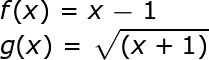### Page 3

#### Question 12 12. Use the graph to find f(f(2)).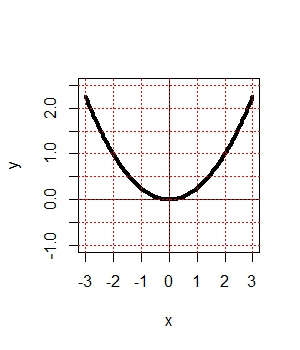#### Question 15 15. The revenue of a company is represented by R(x) and their costs are represented by C(x). What is the profit function R(x) - C(x)?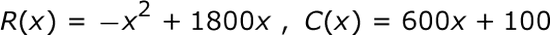### Page 4

#### Question 20 20. Using the graph, find f(f(-2)).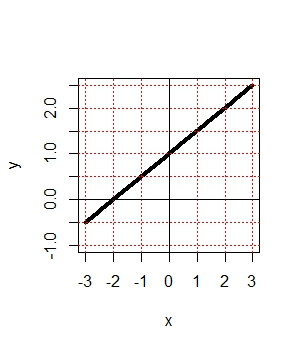### Page 6

#### Question 28 28. In the figure shown below, the graph of the yellow function is a translation of the graph of the blue function. If the expression for the blue function is y(x) = b(x), which of the following is the equation of the yellow function?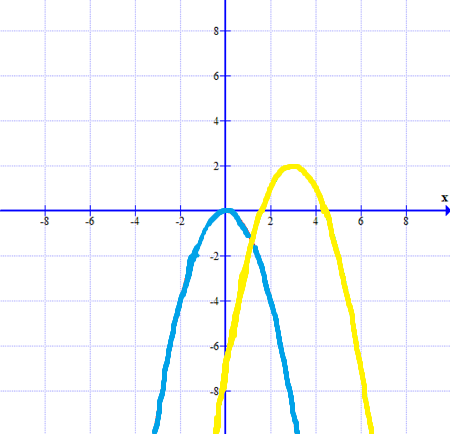#### The Basics of Functions Chapter Exam Instructions

Choose your answers to the questions and click 'Next' to see the next set of questions. You can skip questions if you would like and come back to them later with the yellow "Go To First Skipped Question" button. When you have completed the practice exam, a green submit button will appear. Click it to see your results. Good luck!

Support